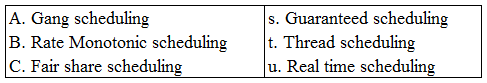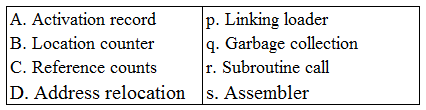# ISRO Scientist or Engineer Computer Science Dec 2017

Instructions

For the following questions answer them individually

Question 61

# Consider the following scheduling :Matching the table in the order A, B, C givesQuestion 62

# A system uses FIFO policy for page replacement. It has 4 page frames with no pages loaded to begin with. The system first accesses 50 distinct pages in some order and then accesses the same 50 pages in reverse order. How many page faults will occur?Question 63

# Which of the following is false?Question 64

# Which of the following is not true with respect to deadlock prevention and deadlock avoidance schemes?Question 65

# Which one of the following are essential features of object oriented language?A. Abstraction and encapsulationB. Strictly-typedC. Type-safe property coupled with sub-type ruleD. Polymorphism in the presence of inheritanceQuestion 66

# Which languages necessarily need heap allocation in the run time environment?Question 67

# Consider the code segmentint i, j, x, y, m, n;n = 20;for (i = 0, i < n; i++){ for (j = 0; j < n; j++) { if (i % 2) { x + = ((4*j) + 5*i); y += (7 + 4*j); } }}m = x + y;Which one of the following is false?Question 68

# Consider the following table :Matching A, B, C, D in the same order gives :Question 69

# Consider a disk sequence with 100 cylinders. The request to access the cylinder occur in the following sequence :4, 34, 10, 7, 19, 73, 2, 15, 6, 20Assuming that the head is currently at cylinder 50, what is the time taken to satisfy all requests if it takes 2 ms to move from one cylinder to adjacent one and shortest seek time first policy is used?Question 70

# A counting semaphore was initialised to 7. Then 20 P (wait) operations and x V (signal) operations were completed on this semaphore. If the final value of semaphore is 5, then the value x will beOR